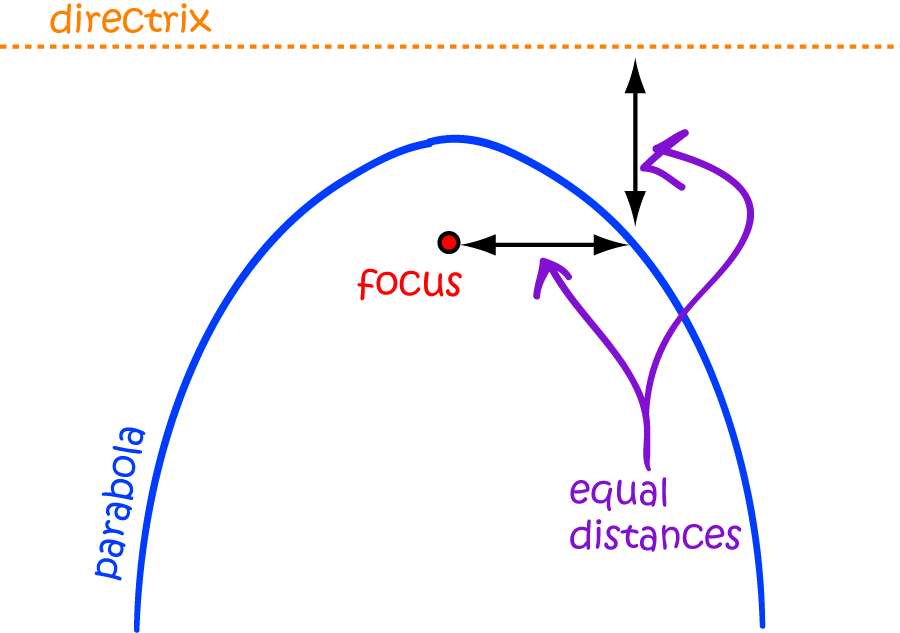# Definition of ParabolaA parabola is a symmetric curve that is shaped like an arch.

Formally, a parabola is the path taken by a point which moves so that its distance from a fixed point, called the focus, is equal to its distance from a fixed line, called the directrix.

For example, the graphs of the following functions are parabolas:

• $y = x^2$
• $y = 4x^2 + 57x + 17$
• $y = -x^2 + 3x + 2$

Along with the circle, ellipse and hyperbola, the parabola is one of the conic sections.

### Description

The aim of this dictionary is to provide definitions to common mathematical terms. Students learn a new math skill every week at school, sometimes just before they start a new skill, if they want to look at what a specific term means, this is where this dictionary will become handy and a go-to guide for a student.

### Audience

Year 1 to Year 12 students

### Learning Objectives

Learn common math terms starting with letter P

Author: Subject Coach
You must be logged in as Student to ask a Question.